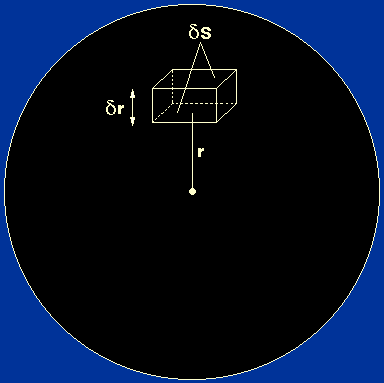The balance between gravity and internal pressure is known as hydrostatic equilibrium. In this section we will derive an equation for this equilibrium condition.

Let us consider the forces acting on a small element of stellar material, as shown in figure 6. The lower face is at a distance r from the centre of the star and the upper face is at a distance r+r. Each of the faces is of areaS. The volume of the element is thereforeSr

and, recalling that mass = density × volume, its mass is given byrSr,

wherer is the density of stellar material at radius r.

 Figure 6: A small element of mass inside a star.There are two forces acting on the element in the radial direction:
• An outward force due to the pressure exerted by the stellar material on the lower face of the element. Note that the forces due to the pressures on the side faces of the element exactly balance.

Recalling that pressure = force / area, this outward force is equal to:

PrS.

• An inward force due to the pressure exerted by the stellar material on the upper face of the element (Pr+r ) and the gravitational attraction of all the stellar material lying within a distance r of the centre of the star. The gravitational force acts as if all of the mass interior to the element were concentrated at the centre of the star and the remainder of the star were neglected.

Recalling that we may write Newton's second law in the form force = mass × acceleration, we obtain the following expression for the inward force:

Pr+rS + (GMr / r2)rSr,

where the mass is given by therSr term and the acceleration is given by GMr / r2.
In hydrostatic equilibrium, the inward force is balanced by the outward force and so we can write:

PrS = Pr+rS + (GMr / r2)rSr.

Rearranging gives:

Pr+r - Pr = - (GMr / r2)rr.

If we are considering an infinitesimal element, we can write,

Pr+r - Pr = (dPr / dr)r            (in the limitr -> 0,Pr /r = dPr / dr, wherePr = Pr+r - Pr ).

By combining these last two equations we obtain:

dPr / dr = - GMrr / r2.

This equation is known as the equation of hydrostatic support. For much of this course, we will omit the subscript r from P, M and, but it is important to remember that these quantities are all functions of r.